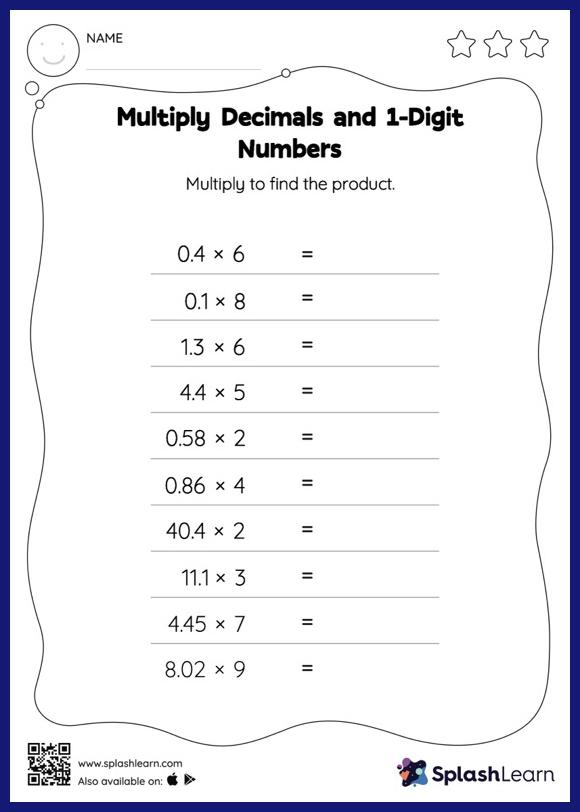# Multiply Decimals and 1-Digit Numbers: Horizontal Multiplication Worksheet

Home > Multiply Decimals and 1-Digit Numbers: Horizontal MultiplicationGive your little one the opportunity to dive deeper into multiplication with this multiply decimals and 1-digit numbers worksheet. Students may omit the decimal point when multiplying a decimal by a one-digit number and then add it when writing the answer. This multiply decimals and 1-digit numbers worksheet gives learners many opportunities to practice this concept.